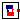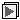## Buildings.Fluid.Storage.BaseClasses

Package with base classes for Buildings.Fluid.Storage

### Information

This package contains base classes that are used to construct the models in Buildings.Fluid.Storage.

Extends from Modelica.Icons.BasesPackage (Icon for packages containing base classes).

### Package Content

NameDescriptionBuoyancy Model to add buoyancy if there is a temperature inversion in the tankIndirectTankHeatExchanger Heat exchanger typically submerged in a fluid with a second fluid circulating through itThirdOrderStratifier Model to reduce the numerical dissipation in a tankExamples Examples for BaseClasses models

##Buildings.Fluid.Storage.BaseClasses.Buoyancy

Model to add buoyancy if there is a temperature inversion in the tank### Information

This model outputs a heat flow rate that can be added to fluid volumes in order to emulate buoyancy during a temperature inversion. For simplicity, this model does not compute a buoyancy induced mass flow rate, but rather a heat flow that has the same magnitude as the enthalpy flow associated with the buoyancy induced mass flow rate.

Extends from Buildings.BaseClasses.BaseIcon (Base icon).

### Parameters

TypeNameDefaultDescription
replaceable package MediumModelica.Media.Interfaces.Pa...Medium model
VolumeV Volume [m3]
IntegernSeg2Number of volume segments
Timetau Time constant for mixing [s]

### Connectors

TypeNameDescription
replaceable package MediumMedium model
HeatPort_aheatPort[nSeg]Heat input into the volumes

### Modelica definition

```model Buoyancy
"Model to add buoyancy if there is a temperature inversion in the tank"
extends Buildings.BaseClasses.BaseIcon;
replaceable package Medium =
Modelica.Media.Interfaces.PartialMedium "Medium model";
parameter Modelica.SIunits.Volume V "Volume";
parameter Integer nSeg(min=2) = 2 "Number of volume segments";
parameter Modelica.SIunits.Time tau(min=0) "Time constant for mixing";

Modelica.Thermal.HeatTransfer.Interfaces.HeatPort_a[nSeg] heatPort
"Heat input into the volumes";

Modelica.SIunits.HeatFlowRate[nSeg-1] Q_flow
"Heat flow rate from segment i+1 to i";
protected
parameter Medium.ThermodynamicState sta_default = Medium.setState_pTX(T=Medium.T_default,
p=Medium.p_default, X=Medium.X_default[1:Medium.nXi]);
parameter Modelica.SIunits.Density rho_default=Medium.density(sta_default)
"Density, used to compute fluid mass";
parameter Modelica.SIunits.SpecificHeatCapacity cp_default=Medium.specificHeatCapacityCp(sta_default)
"Specific heat capacity";
parameter Real k(unit="W/K") = V*rho_default*cp_default/tau/nSeg
"Proportionality constant, since we use dT instead of dH";
Modelica.SIunits.TemperatureDifference dT[nSeg-1]
equation
for i in 1:nSeg-1 loop
dT[i] = heatPort[i+1].T-heatPort[i].T;
Q_flow[i] = k*noEvent(smooth(1, if dT[i]>0 then dT[i]^2 else 0));
end for;

heatPort.Q_flow = -Q_flow;
for i in 2:nSeg-1 loop
heatPort[i].Q_flow = -Q_flow[i]+Q_flow[i-1];
end for;
heatPort[nSeg].Q_flow = Q_flow[nSeg-1];
end Buoyancy;
```

##Buildings.Fluid.Storage.BaseClasses.IndirectTankHeatExchanger

Heat exchanger typically submerged in a fluid with a second fluid circulating through it### Information

This model is a heat exchanger with a moving fluid on one side and a stagnant fluid on the other. It is intended for use when a heat exchanger is submerged in a stagnant fluid. For example, the heat exchanger in a storage tank which is part of a solar thermal system.

This component models the fluid in the heat exchanger, convection between the fluid and the heat exchanger, and convection from the heat exchanger to the surrounding fluid.

The fluid ports are intended to be connected to a circulated heat transfer fluid while the heat port is intended to be connected to a stagnant fluid.

Extends from Buildings.Fluid.Interfaces.TwoPortFlowResistanceParameters (Parameters for flow resistance for models with two ports), Buildings.Fluid.Interfaces.LumpedVolumeDeclarations (Declarations for lumped volumes), Buildings.Fluid.Interfaces.PartialTwoPortInterface (Partial model transporting fluid between two ports without storing mass or energy).

### Parameters

TypeNameDefaultDescription
replaceable package MediumHexModelica.Media.Interfaces.Pa...Heat transfer fluid flowing through the heat exchanger
replaceable package MediumPartialMediumMedium in the component
IntegernSeg Number of segments in the heat exchanger
HeatCapacityCHex Capacitance of the heat exchanger [J/K]
VolumevolHexFlu Volume of heat transfer fluid in the heat exchanger [m3]
DiameterdExtHex Exterior diameter of the heat exchanger pipe [m]
Nominal condition
Pressuredp_nominal Pressure [Pa]
MassFlowRatem_flow_nominal Nominal mass flow rate [kg/s]
HeatFlowRateQ_flow_nominal Heat transfer at nominal conditions [W]
TemperatureTTan_nominal Temperature of fluid inside the tank at UA_nominal [K]
TemperatureTHex_nominal Temperature of fluid inside the heat exchanger at UA_nominal [K]
Realr_nominal0.5Ratio between coil inside and outside convective heat transfer
Initialization
MassFlowRatem_flow.start0Mass flow rate from port_a to port_b (m_flow > 0 is design flow direction) [kg/s]
Pressuredp.start0Pressure difference between port_a and port_b [Pa]
Flow resistance
BooleancomputeFlowResistancetrue=true, compute flow resistance. Set to false to assume no friction
Booleanfrom_dpfalse= true, use m_flow = f(dp) else dp = f(m_flow)
BooleanlinearizeFlowResistancefalse= true, use linear relation between m_flow and dp for any flow rate
RealdeltaM0.1Fraction of nominal flow rate where flow transitions to laminar
Dynamics
Equations
DynamicsenergyDynamicsModelica.Fluid.Types.Dynamic...Formulation of energy balance
DynamicsmassDynamicsenergyDynamicsFormulation of mass balance
Initialization
AbsolutePressurep_startMedium.p_defaultStart value of pressure [Pa]
TemperatureT_startMedium.T_defaultStart value of temperature [K]
MassFractionX_start[Medium.nX]Medium.X_defaultStart value of mass fractions m_i/m [kg/kg]
ExtraPropertyC_start[Medium.nC]fill(0, Medium.nC)Start value of trace substances
ExtraPropertyC_nominal[Medium.nC]fill(1E-2, Medium.nC)Nominal value of trace substances. (Set to typical order of magnitude.)
Assumptions
BooleanallowFlowReversalsystem.allowFlowReversal= true to allow flow reversal, false restricts to design direction (port_a -> port_b)
MassFlowRatem_flow_small1E-4*abs(m_flow_nominal)Small mass flow rate for regularization of zero flow [kg/s]
BooleanhomotopyInitializationtrue= true, use homotopy method
Diagnostics
Booleanshow_Tfalse= true, if actual temperature at port is computed

### Connectors

TypeNameDescription
replaceable package MediumHexHeat transfer fluid flowing through the heat exchanger
FluidPort_aport_aFluid connector a (positive design flow direction is from port_a to port_b)
FluidPort_bport_bFluid connector b (positive design flow direction is from port_a to port_b)
HeatPort_aport[nSeg]Heat port connected to water inside the tank

### Modelica definition

```model IndirectTankHeatExchanger
"Heat exchanger typically submerged in a fluid with a second fluid circulating through it"

replaceable package MediumHex = Modelica.Media.Interfaces.PartialMedium
"Heat transfer fluid flowing through the heat exchanger";

extends Buildings.Fluid.Interfaces.TwoPortFlowResistanceParameters;
extends Buildings.Fluid.Interfaces.LumpedVolumeDeclarations;
extends Buildings.Fluid.Interfaces.PartialTwoPortInterface(
showDesignFlowDirection=false,
final show_T=false);

parameter Integer nSeg(min=2) "Number of segments in the heat exchanger";
parameter Modelica.SIunits.HeatCapacity CHex
"Capacitance of the heat exchanger";
parameter Modelica.SIunits.Volume volHexFlu
"Volume of heat transfer fluid in the heat exchanger";
parameter Modelica.SIunits.HeatFlowRate Q_flow_nominal
"Heat transfer at nominal conditions";

final parameter Modelica.SIunits.ThermalConductance UA_nominal=
abs(Q_flow_nominal/(THex_nominal-TTan_nominal))
"Nominal UA value for the heat exchanger";
parameter Modelica.SIunits.Temperature TTan_nominal
"Temperature of fluid inside the tank at UA_nominal";
parameter Modelica.SIunits.Temperature THex_nominal
"Temperature of fluid inside the heat exchanger at UA_nominal";
parameter Real r_nominal(min=0, max=1)=0.5
"Ratio between coil inside and outside convective heat transfer";

parameter Modelica.SIunits.Diameter dExtHex
"Exterior diameter of the heat exchanger pipe";

parameter Modelica.Fluid.Types.Dynamics energyDynamics=Modelica.Fluid.Types.Dynamics.DynamicFreeInitial
"Formulation of energy balance";
parameter Modelica.Fluid.Types.Dynamics massDynamics=energyDynamics
"Formulation of mass balance";

Modelica.Thermal.HeatTransfer.Interfaces.HeatPort_a port[nSeg]
"Heat port connected to water inside the tank";

FixedResistances.FixedResistanceDpM res(
redeclare final package Medium = Medium,
final dp_nominal=dp_nominal,
final m_flow_nominal=m_flow_nominal,
final allowFlowReversal=allowFlowReversal,
final homotopyInitialization=homotopyInitialization,
final show_T=show_T,
final from_dp=from_dp,
final linearized=linearizeFlowResistance)
"Calculates the flow resistance and pressure drop through the heat exchanger";

MixingVolumes.MixingVolume vol[nSeg](each nPorts=2,
each m_flow_nominal=m_flow_nominal,
redeclare each package Medium = MediumHex,
each V=volHexFlu/nSeg,
each energyDynamics=energyDynamics,
each massDynamics=massDynamics,
each p_start=p_start,
each T_start=T_start,
each X_start=X_start,
each C_start=C_start,
each C_nominal=C_nominal) "Heat exchanger fluid";
Modelica.Thermal.HeatTransfer.Components.HeatCapacitor Cap[nSeg](each C=CHex/
nSeg, T(each start=T_start)) if
"Thermal mass of the heat exchanger";
protected
Sensors.MassFlowRate senMasFlo(redeclare package Medium = MediumHex)
"Mass flow rate of the heat transfer fluid";
Modelica.Thermal.HeatTransfer.Components.Convection htfToHex[nSeg]
"Convection coefficient between the heat transfer fluid and heat exchanger";
Modelica.Thermal.HeatTransfer.Components.Convection HexToTan[nSeg]
"Convection coefficient between the heat exchanger and the surrounding medium";
Modelica.Thermal.HeatTransfer.Sensors.TemperatureSensor
temSenHex[nSeg]
"Temperature of the heat transfer fluid";
Modelica.Thermal.HeatTransfer.Sensors.TemperatureSensor temSenWat[nSeg]
"Temperature sensor of the fluid surrounding the heat exchanger";
Modelica.Blocks.Routing.Replicator rep(nout=nSeg)
"Replicates senMasFlo signal from 1 seg to nSeg";
HeatExchangers.BaseClasses.HACoilInside hAPipIns[nSeg](
each m_flow_nominal=m_flow_nominal,
each hA_nominal=UA_nominal/nSeg*(r_nominal + 1)/r_nominal,
each T_nominal=THex_nominal)
"Computation of convection coefficients inside the coil";
HeatExchangers.BaseClasses.HANaturalCylinder hANatCyl[nSeg](
redeclare each final package Medium = Medium,
each final ChaLen=dExtHex,
each final hA_nominal=UA_nominal/nSeg*(1 + r_nominal),
each final TFlu_nominal=TTan_nominal,
each final TSur_nominal=TTan_nominal-(r_nominal/(1+r_nominal))*(TTan_nominal-THex_nominal))
"Calculates an hA value for each side of the heat exchanger";
Modelica.Thermal.HeatTransfer.Sensors.TemperatureSensor temSenSur[nSeg]
"Temperature at the external surface of the heat exchanger";

equation
for i in 1:(nSeg - 1) loop
connect(vol[i].ports, vol[i + 1].ports);
end for;

connect(rep.u,senMasFlo. m_flow);
connect(port,HexToTan. fluid);
connect(vol.ports,senMasFlo. port_b);
connect(Cap.port,HexToTan. solid);
connect(vol.heatPort,htfToHex. fluid);
connect(htfToHex.solid,HexToTan. solid);
connect(temSenHex.T, hAPipIns.T);
connect(hAPipIns.hA, htfToHex.Gc);
connect(HexToTan.solid,temSenSur. port);
connect(temSenWat.port, port);
connect(temSenSur.T, hANatCyl.TSur);
connect(hANatCyl.TFlu, temSenWat.T);
connect(port_a, senMasFlo.port_a);
connect(vol[nSeg].ports, res.port_a);
connect(res.port_b, port_b);
connect(temSenHex.port, vol.heatPort);
connect(rep.y, hAPipIns.m_flow);
connect(hANatCyl.hA,HexToTan. Gc);
end IndirectTankHeatExchanger;
```

##Buildings.Fluid.Storage.BaseClasses.ThirdOrderStratifier

Model to reduce the numerical dissipation in a tank### Information

This model reduces the numerical dissipation that is introduced by the standard first-order upwind discretization scheme which is created when connecting fluid volumes in series.

The model is used in conjunction with Modelica.Fluid.Storage.Stratified. It computes a heat flux that needs to be added to each volume of Modelica.Fluid.Storage.Stratified in order to give the results that a third-order upwind discretization scheme (QUICK) would give.

The QUICK method can cause oscillations in the tank temperatures since the high order method introduces numerical dispersion. There are two ways to reduce the oscillations:

• To use an under-relaxation coefficient `alpha` when adding the heat flux into the volume.
• To use the first-order upwind for `hOut` and `hOut[nSeg]`. Note: Using it requires `nSeg>=4`.

Both approaches are implemented in the model.

The model is used by Buildings.Fluid.Storage.StratifiedEnhanced.

#### Limitations

The model requires at least 4 fluid segments. Hence, set `nSeg` to 4 or higher.

Extends from Buildings.BaseClasses.BaseIcon (Base icon).

### Parameters

TypeNameDefaultDescription
replaceable package MediumModelica.Media.Interfaces.Pa...Medium model
MassFlowRatem_flow_small Small mass flow rate for regularization of zero flow [kg/s]
IntegernSeg Number of volume segments
Realalpha0.5Under-relaxation coefficient (1: QUICK; 0: 1st order upwind)

### Connectors

TypeNameDescription
replaceable package MediumMedium model
HeatPort_aheatPort[nSeg]Heat input into the volumes
input RealInputm_flowMass flow rate from port a to port b
input RealInputH_flow[nSeg + 1]Enthalpy flow between the volumes
FluidPort_afluidPort[nSeg + 2]Fluid port, needed to get pressure, temperature and species concentration

### Modelica definition

```model ThirdOrderStratifier
"Model to reduce the numerical dissipation in a tank"
extends Buildings.BaseClasses.BaseIcon;
replaceable package Medium = Modelica.Media.Interfaces.PartialMedium
"Medium model";

parameter Modelica.SIunits.MassFlowRate m_flow_small(min=0)
"Small mass flow rate for regularization of zero flow";
parameter Integer nSeg(min=4) "Number of volume segments";

parameter Real alpha(
min=0,
max=1) = 0.5 "Under-relaxation coefficient (1: QUICK; 0: 1st order upwind)";

Modelica.Thermal.HeatTransfer.Interfaces.HeatPort_a[nSeg] heatPort
"Heat input into the volumes";

Modelica.Blocks.Interfaces.RealInput m_flow
"Mass flow rate from port a to port b";

Modelica.Blocks.Interfaces.RealInput[nSeg + 1] H_flow
"Enthalpy flow between the volumes";

Modelica.Fluid.Interfaces.FluidPort_a[nSeg + 2] fluidPort(redeclare each
package Medium = Medium)
"Fluid port, needed to get pressure, temperature and species concentration";

protected
Modelica.SIunits.SpecificEnthalpy[nSeg + 1] hOut
"Extended vector with new outlet enthalpies to reduce numerical dissipation (at the boundary between two volumes)";
Modelica.SIunits.SpecificEnthalpy[nSeg + 2] h
"Extended vector with port enthalpies, needed to simplify loop";
Modelica.SIunits.HeatFlowRate Q_flow[nSeg]
"Heat exchange computed using upwind third order discretization scheme";
//    Modelica.SIunits.HeatFlowRate Q_flow_upWind
//     "Heat exchange computed using upwind third order discretization scheme"; //Used to test the energy conservation
Real sig
"Sign used to implement the third order upwind scheme without triggering a state event";
Real comSig
"Sign used to implement the third order upwind scheme without triggering a state event";

equation
assert(nSeg >= 4, "
Number of segments of the enhanced stratified tank should be no less than 4 (nSeg>=4).");

// assign zero flow conditions at port
fluidPort[:].m_flow = zeros(nSeg + 2);
fluidPort[:].h_outflow = zeros(nSeg + 2);
fluidPort[:].Xi_outflow = zeros(nSeg + 2, Medium.nXi);
fluidPort[:].C_outflow = zeros(nSeg + 2, Medium.nC);

// assign extended enthalpy vectors
for i in 1:nSeg + 2 loop
h[i] = inStream(fluidPort[i].h_outflow);
end for;

// Value that transitions between 0 and 1 as the flow reverses.
sig = Modelica.Fluid.Utilities.regStep(
m_flow,
1,
0,
m_flow_small);
// at surface between port_a and vol1

comSig = 1 - sig;

// at surface between port_a and vol1
hOut = sig*h + comSig*h;
// at surface between vol[nSeg] and port_b
hOut[nSeg + 1] = sig*h[nSeg + 1] + comSig*h[nSeg + 2];

// Pros: These two equations can further reduce the temperature overshoot by using the upwind
// Cons: The minimum of nSeg hase to be 4 instead of 2.
hOut = sig*h + comSig*h;
// at surface between vol1 and vol2
hOut[nSeg] = sig*h[nSeg] + comSig*h[nSeg + 1];
// at surface between vol[nSeg-1] and vol[nSeg]

for i in 3:nSeg - 1 loop
// at surface between vol[i-1] and vol[i]
// QUICK method
hOut[i] = 0.5*(h[i] + h[i + 1]) - comSig*0.125*(h[i + 2] + h[i] - 2*h[i + 1])
- sig*0.125*(h[i - 1] + h[i + 1] - 2*h[i]);
//     hOut[i] = 0.5*(h[i]+h[i+1]); // Central difference method
end for;

for i in 1:nSeg loop
// difference between QUICK and UPWIND; index of H_flow is same as hOut
Q_flow[i] = m_flow*(hOut[i + 1] - hOut[i]) - (H_flow[i + 1] - H_flow[i]);
end for;

//   Q_flow_upWind = sum(Q_flow[i] for i in 1:nSeg); //Used to test the energy conservation

for i in 1:nSeg loop
// Add the difference back to the volume as heat flow. An under-relaxation is needed to reduce
// oscillations caused by high order method
heatPort[i].Q_flow = Q_flow[i]*alpha;
end for;
end ThirdOrderStratifier;
```

Automatically generated Thu Oct 24 15:10:03 2013.